MATLAB Tutorial "Fundamentals"

MATLAB is a commercial computing environment developed by MathWorks, targeted specifically at numerical computations and simulations, that uses a proprietary language, also called MATLAB. MATLAB offers functionality for creating and manipulating arrays and matrices, for plotting functions and data and for implementing mathematical algorithms that solve physical and engineering problems. This tutorial gives an introduction to the MATLAB environment and language as needed by engineers for learning Numerical Methods. Basic MATLAB syntax (variables, input, output, vectors, matrices, functions, plotting) is illustrated using small examples that are saved as MATLAB scripts. All examples will also run in the open source software Octave.

Motivation

A computing environment like MATLAB or Octave offers a broad support for solving mathematical problems analytically as well as numerically. MATLAB programs hide implementation details and much of the underlying complexity. Engineers can set up models and generate solutions even without understanding mathematical model or numerical method very well initially, so they can just focus on their physical problem. Since powerful solvers are available, the focus is shifted from solving a problem to modeling it correctly.

MATLAB has powerful support for solving numerical problems that model engineering problems. For example, MATLAB's Ordinary Differential Equation solvers solve time-dependent problems with a variety of properties. The PDE (Partial Differential Equation) Toolbox provides functions for solving partial differential equations that model structural mechanics and heat transfer problems using finite element analysis. All functions are well-documented and there are many examples available.

Octave is a computing environment and scientific programming language very similar with MATLAB and the open source pendant of MATLAB. While the environment is not as sleek as MATLAB, it is free, has the same basic capabilities and is suited for learning MATLAB language basics.

Overview

The tutorial is structured in nine sections that explain usage of the MATLAB Integrated Development Environment and the most important MATLAB commands:

Further learning materials include a YouTube Video and two MATLAB Quizzes. The YouTube-Video gives additional insight in how to learn MATLAB Fundamentals using this tutorial in a step-by-step way.

Environments: MATLAB and Octave

In order to run MATLAB scripts, a MATLAB installation is required, a test version is downloadable from the Mathworks website. Alternatively, you can download and install Octave, which is free. In the following we use the term integrated development environments (IDE) and refer to "MATLAB IDE" and "Octave IDE". After installation, the IDE's should look similarly as in the screenshots below.

In MATLAB, the default window layout displays file browser, workspace, editor and command window in a 4x4 grid, and be configured and aligned differently using the Layout menu.

In Octave, the default window layout organizes file browser, workspace and command history in the left part of the main view and command window, documentation, editor and variable editor in the right part of the main view. You can see either the command window or the editor, but not both at the same time, which is an inconvenience. When running scripts, it is often required to see editor and output next to each other. The only way to do this, is by undocking the command window, resizing it, and placing it on top of the main window.

MATLAB EnvironmentMATLAB's Editor-Tab is organized in four windows, that are used for developing and running scripts.

• File browser (top-left): displays the folders that contain m-files. Here you create new folders and new scripts.
• Workspace (bottom-left): displays the variables and functions of the running scripts.
• Editor (top-right): displays the contents of the script files. The actual programming is done in the editor.
• Command Window (bottom-right): displays commands and the output of statements.

Octave EnvironmentOctave IDE similarly is organized in five main windows, that are used for developing and running scripts.

• File Browser: displays the folders that contain m-files. Here you create new folders and new scripts.
• Workspace: displays the variables and functions of the running scripts.
• Command history: displays the history of commands. Can be cleared by using history -c.
• Editor: displays the contents of the script files. The actual programming is done in the editor.
• Command Window: displays commands and the output of statements.

A MATLAB program can be created in different ways:

• Interactive mode:
MATLAB can be used in interactive mode, by entering commands directly in the command window. Output is also shown in the command window. Interactive mode is useful for tutorials and for testing small program parts.
• MATLAB Script:
Larger MATLAB programs are collections of MATLAB scripts stored in a common folder. The folder can be anywhere on your computer but must be added to the MATLAB path by using HOME > Set Path Menu.
• MATLAB LiveScript:
MATLAB LiveScripts are a special type of scripts with extended documentation features. While an .m-file contains source code and comments and displays output in the command window and figures in the figure editor, a LiveScript (.mlx file) offers more functionality. In a LiveScript, user input, source code and output including graphics are displayed in one single interactive window. The effect of a change in the source code can be seen directly and immediately in the output results (graphics, text output, etc.). While a pure code script is more efficient when the task is to solve a specific problem, MATLAB LiveScripts can be used as teaching tool and for demonstration purpose, since they convey a better understanding of the steps, commands and functions used to solve a problem.

A MATLAB script is a text file with the extension .m, that contains the statements and comments of the program. MATLAB script names may contain only letters, numbers and subscript. Valid names are for example myscript1.m, myscript2.m, myfun1.m, myfun2.m.
MATLAB scripts are created using the menu items in the EDITOR > FILE menu (New, Open, Save etc.) and are executed using the menu items in the EDITOR > RUN-Menu (Run, Run and Advance, Run Section).

First Lines in MATLAB

MATLAB statements are expressions or assignments, variable declarations , operations with variables, input- or output-instructions, control flow statements or function calls, that perform specific tasks (plots, mathematical computations etc.). MATLAB statements can be terminated with a semicolon or not. If terminated with a semicolon, a statement will not generate any output in the command window.
Before starting to explore the MATLAB language, it is useful to learn some commands that are needed frequently to keep a clean and organized workspace and command window.

• clear – Clears workspace variables. You can delete selected variables or all variables. Useful for example when you run multiple scripts that use the same variable names.
• clc – Clears command window (instructions and output). Useful when you want to start over with a clean slate.
• format compact – Compactifies the command window output. Another useful format command is format long, that is used to display floating point numbers in the command window with double precision.
• help – If you type help followed by the name of a command or function, the corresponding help page is displayed.

Example
Find out what the clc command does.

When typing "help clc" in the command window, the documentation for the clc command is shown as depicted. By clicking the displayed "Reference page ... "-link, the reference page for the command in the MATLAB online documentation is opened, with the complete information and examples on how to use the command.

Output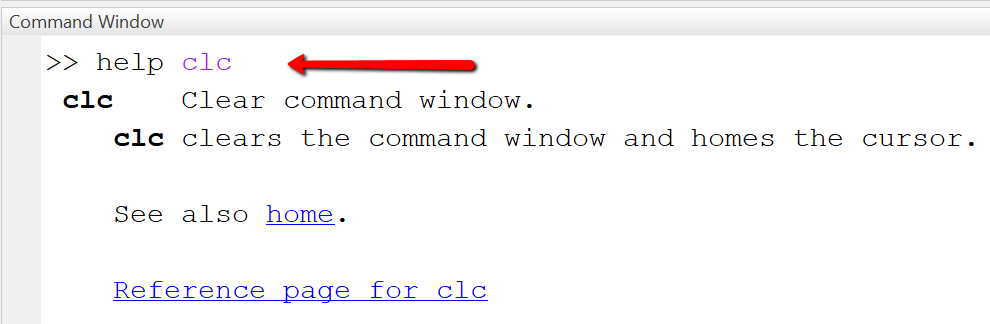"Hello World" Program in MATLAB

A "Hello World" program is the smallest executable program in a programming language and prints the text "Hello World" to default output (here: the command window). We do it first in interactive mode and then in a script.

Interactive mode

In interactive mode, we use only the command window. Statements are entered next to the the command prompt (indicated by >>) and executed by pressing ENTER.

Example
Using interactive mode and the command window.

Type the statement text='Hello World' in the command window and then press ENTER. As shown in the picture, the variable (here: text) and its content (here: Hello World) are shown as output in the command window. Next, type the statement disp('Hello World') and press ENTER. Again, the string is displayed, but this time without the name of the variable.

Output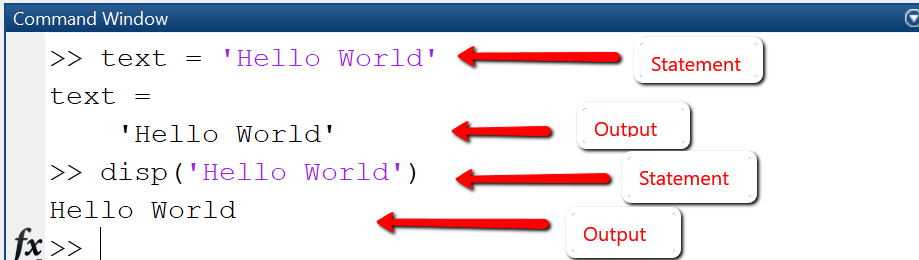Script mode

In script mode, we first create a new script with the name "test_hello.m", then type the MATLAB statements below in the script file and save it, and finally execute the script by pressing the "RUN"-Button.

Example
Using script files to store larger programs.

The code starts in line 1 with a comment (indicated by "%"-symbol), that states the name of the script file. Note that the following lines are also commented. In lines 2 and 3 we insert code for resetting / preparing workspace and command window. In line 4 we create a new variable "text" and assign it the value 'Hello world'. In line 5 we print the string 'Hello World' using disp().

% Script: test_hello.m
clear;clc; % Clear workspace and command window
format compact % Compactify command window output
text = 'Hello World'
disp('Hello World!');
Output

The MATLAB platform after creating, editing, saving and running the script test_hello.m looks as shown. In the menu bar we have highlighted the New, Save and Run menu items, that have been used to create the new script, save, and execute it in turn. Top-right displays the editor with the code of the script test_hello.m and bottom-right the command window with the output after executing the script.Comments in MATLAB start with a "%"-symbol followed by text. Comments are used for documentation purpose, they are not executed by the system. Two percent-symbols "%%" mark a section. Sections can be executed separately using the RUN SECTION-Button.

%% Section 1
% Comment 1: This is the first comment for section 1.
text = 'Let''s start simple' % Here we assign a value to the variable named text
%% Section 2
% Comment 2: This is the first comment for section 2.
a = 10 % Here we assign a value to variable a
b = 20 % Here we assign a value to variable b

Variables and data types

Variables are named memory spaces that store the data of your program. MATLAB variables are declared by simply assigning a value to them. The data type (number, string, array etc.) is assigned automatically by MATLAB. For example, all numbers (integer, floating points) are stored internally as double precision floating point numbers. Knowing the data type is important, since the data type determines the set of operations that you can perform with your variables. For example, you can add two numbers or concatenate two strings with the "+"-operation as in the example below, but it is not possible to add a number to a string: sum = a + " apples" is an invalid statement. Before appending a number to a string, it must be converted to a string using num2string: sum = num2string(a) + " apples" is a valid statement.

Example
Using variables: declaration and operations

The example shows how to declare variables (numerical and string) and perform operations on them. Note that the +-symbol denotes different operations: in line 3, the addition of numbers, in line 5, the concatenation of strings.

% test_variables.m
a = 10; b = 20.5;
sum = a + b
s1 = "apples";s2 = "pears";
text1 = s1 + "+" + s2
text2 = replace(text1, '+',' or ')
Output

When executing the script test_variables.m, output in command window is as shown.Code explanation:

• Line 2: Declare two variables a, b by assigning values to them. Since the declarations are terminated with semicolon, output of the variables to the command window is suppressed.
• Line 3: Add the two variables (actually, their values) and store the result in the variable sum.
• Line 4: Declare two string variables s1, s2.
• Line 5: Concatenate the strings to form a new string.
• Line 6: Replace the "+"-character with the string ' or '.

Data types

MATLAB supports different data types, that are represented by classes:

• Numerical data types: int, single, double
• Boolean data types: logical
• String data types: string, char

In order to find out the exact data type and size of your variables, use the commands whos, class. With whos, you can find out size and type of workspace objects. With class, you can find out the data type of a variable.

Example
Declare variables and find out their data type.

We declare twos variable a and b by assigning numeric values, 10 and 20.5 respectively. MATLAB assumes data type double even when you assign an integer number and accordingly reserves 8 Byte. MATLAB also considers a variable to be matrix with one row and one column, this is why the size is shown as 1x1.

a = 10;
b = 20.5;
whos a
whos b
Output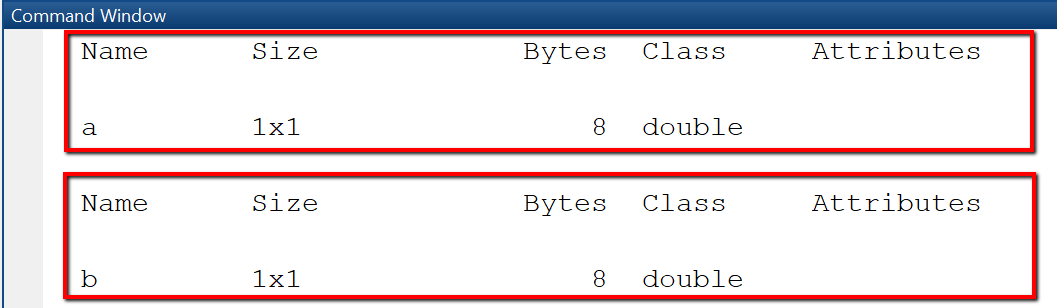String variables

String variables in MATLAB can be either of class "string" or of class "character array". When you create a string using single quotes, it is stored as character array. When you create a string using double quotes, it is stored as a string. A character array is a sequence of characters, just as a numeric array is a sequence of numbers. The String class provide a set of functions for working with text as data.

Example
Declare string variables and find out their data type.
text1 = "Hello"
whos text1
text2 = 'from Kaiserslautern'
whos text2
OutputInput and Output-Commands

Data stored in variables can either be viewed in the workspace or displayed in the command window. While the workspace shows the raw variable values, the command window is used for formatted output.

Output to command window

There are three ways to output variable values to command window.

• Type the name of a variable in a new line, with no terminating semicolon. In an assignment statement, omit semicolon at the end of a statement. The content of the variable then is displayed in the command window.
• Use disp()-function. This function takes as argument a variable / vector / matrix and displays it, omitting the name of the variable.
• Use fprintf()-function. This function builds a formatted output by using placeholders for the variables to be inserted. In our example, the value of variable a will be inserted in the place indicated by the first "%d", the value of variable b will be inserted in the place indicated by the second "%d" etc.
Example: Output to command window
Different ways to create output to command window.

In this example we calculate the sum of two variables and generate output in the command window.

% test_output.m
a = 10; b = 20; sum = a + b;
% (1) No semicolon
disp('(1) Output by typing variable name')
sum % output: sum = 30
% (2) Use disp
disp('(2) Output using disp()')
disp([a b sum]) % output: 10 20 30
% (3) Use fprintf for formatted output
disp('(3) Output using fprintf')
% output: a = 10, b = 20, Sum = 30
fprintf('a = %d, b = %d, Sum = %d\n', a, b, sum)
Output

When running the script test_output.m, output looks as displayed.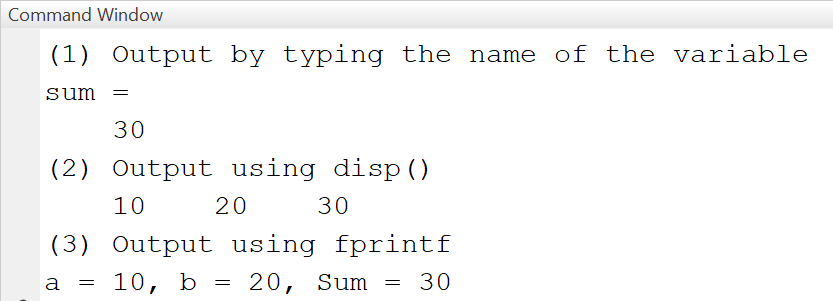Input from command window

When presenting a MATLAB script to non-technical users, it may be helpful to let them enter configuration parameters as input from the command window or an input dialog. Input entered in the command window can be stored in variables using the input(prompt)-function.

Example
Input using prompt.
% Display a prompting text
prompt = 'Enter a: ';
a = input(prompt);
prompt = 'Enter b: ';
b = input(prompt);
sum = a + b;
fprintf("Sum = %.2f\n", sum);
OutputInput dialog

An input dialog as shown below is created using the MATLAB-function inputdlg. We pass four arguments to the function:

• prompt -- the labels of the input fields
• dlgtitle -- the title of the dialog
• windowsize -- the size of the window
• definput -- specifies the default value for each edit field. The definput argument must contain the same number of elements as prompt.

The function returns an array of strings "answer", that contains the values entered in the edit fields.

Example
Input dialog for two variables.
prompt = {'Enter a:', 'Enter b:'};
dlgtitle = 'Input';
windowsize = [1 50];
definput = {'10','20'};
answer = inputdlg(prompt, dlgtitle, windowsize, definput)
OutputInput from files

Many applications require to read tabular data from *.txt, *.csv or *.xlsx files, or binary data (images). For this type of requirement, MATLAB offers functions such as inputdata, readtable and load. They all require as input a file, specified by its name including the path, and return an internal representation of the data, either as table or as struct or array. If only the filename is specified, MATLAB searches for a file in the same folder where the script is placed. Alternatively, it is possible to specify the full path to the file, for example 'C:\temp\accidents.csv'. Note that when importing from Excel, the row and column headers should be specified correctly. Also, it is important to use the correct delimiters for the decimal point, in a computer with German settings, this will be comma (,), else dot (.).

• A = importdata(filename, delimiter) loads data into array A. This function is used for importing tabular data as well as images.
• T = readtable(filename, options) reads tabular data from a file and stores it internally in a table object. This function is useful when you have spreadsheet-like data as in an excel-sheet, where each column has its own data type. Column headers are imported (or not) by specifying the options 'ReadVariableNames' and 'PreserveVariableNames'.
• T = load(filename) loads variables or data from a file into workspace: if the file is a *.mat-file, then in loads the variables from this file, else if it is a text-file, it loads the data into a double-precision array.

When developing a numerical method, it is often required to convert the table object returned by importdata into an array or extract single columns or rows of the imported table into a column vector. This is done by using the function table2array as in the next example.

Example: Importing data from files

For this example we use two input files, accidents_5.csv and accidents_5.xlsx, that we store in the same folder as our test script. The csv-file contains number-only entries separated with semicolon and has no headers. The Excel-file contains the same data as the csv-file, but with headers.

% (1) Load data using importdata
A1 = importdata('accidents_5.csv', ';')
% Convert the table into an array
A = table2array(T(2:end, 1:end))
% Extract the second row
row2 = A(2,:)

%% Find out the type of the returned objects
whos T % It's a table
whos A1 % It's an array
whos A2 % It's an array
Output in the command window
The import stores data as array or table objects
A1 =
1      493782         164
2      572059          43
3      608827          98
4      626932         101
5      642200         100
T =
5×3 table
Nr    Population    Accidents
__    __________    _________
1     4.9378e+05       164
2     5.7206e+05        43
3     6.0883e+05        98
4     6.2693e+05       101
5      6.422e+05       100
A =
2      572059          43
3      608827          98
4      626932         101
5      642200         100
row2 =
3      608827          98
A2 =
1      493782         164
2      572059          43
3      608827          98
4      626932         101
5      642200         100

Control flow: Conditional statements and loops

Control flow statements are used to determine which block of code to execute at run time (conditional statements, if-else) or to execute a block of code repeatedly (while-loop, for-loop).

Conditional statements (if-else)

Conditional statements are used to control which of two or more statement blocks are executed depending on a condition. In MATLAB, as in most programming languages, they are implemented using the if-else-syntax.

Example
Show message according to the size of the number

In this example the user is asked to enter a number smaller that 100 and according to the size of the input a message is displayed. In line 5, the condition "a < 30" is evaluated: If true, the statement in line 5 is executed and the program flow continues in the first line after the if-else-statement.

% test_ifelse.m
prompt = 'Enter number < 100: ';
a = input(prompt);
disp('Your number:'); disp(a); % display it
if a < 30
disp('Small number!')
elseif a < 80
disp('Medium number!')
else
disp('Large number!')
end
Output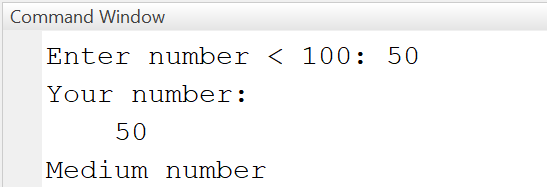Loops

Loops execute a block of code repeatedly as long as a condition is satisfied. MATLAB has two types of loops: while and for.

While loop
A while loop allows statements to be executed repeatedly, as long as an execution condition is met. The variable that is queried in the condition is not automatically increased, so it must be explicitly incremented in the body of the loop. If there is no increase in the variable, the loop is executed endlessly.

Example: While-Loop
Calculate sum of first n numbers: sum = 1+2+...+n

In this example, a counter variable i is initialized with 1. In line 3 the loop condition "i <= 5" is checked. If "true", the statements in line 4-6 are executed (print the value of i, add the value of i to the value of sum, increment the value of i) and then the loop condition is checked again and the loop is repeated.

sum = 0;
i = 1; % (1) Initialize counter i
while i <= 5 % (2) Check condition
fprintf("%d + \n", i);
sum = sum + i;
i = i+1; % (3) Increment counter
end
Output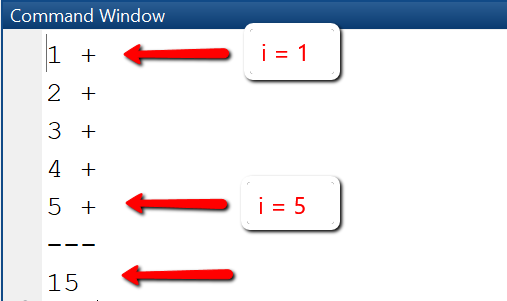For loop
A for loop is a counting loop that defines a start and end condition for a counting variable (loop counter) and repeats a statement or a group of statements for a number of loop passes a specified by the loop counter. The loop counter is increased by 1 (or another step size) after each loop pass.

Example: For loop
Calculate sum of first n numbers: sum = 1+2+...+n

In this example, a counter variable i is initialized with 1. In line 3 the loop condition "i <= 5" is checked. If "true", the statements in line 4-6 are executed (print the value of i, add the value of i to the value of sum, increment the value of i) and then the loop condition is checked again and the loop is repeated.

n = 6; sum = 0;
% Start at index 1, use increment 2, end at index n
for i=1:2:n
fprintf("%d + \n", i);
sum = sum + i;
end
fprintf("---\n");
fprintf("%d\n", sum);
Output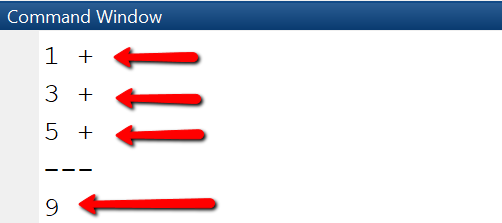Vectors

Vectors are one-dimensional arrays that store information. They can be stored in row or column format. For example, x = [1 4 9] will be a row vector, and x = [1; 4; 9] will be a column vector. Vectors are created in different ways: by listing their elements explicitly in square brackets, using functions, or using for-loops.
Elements of a vector are accessed using an index that starts at 1. For example, to access element i in vector x, we write x(i).

Example: Vectors
Create vector with 5 elements

We first create a row vector with 5 elements, display the second element of the vector, then the elements 2, 3 and 4 and change element 3 to be the sum of the first two elements.

x = [1 4 9 16 25]
disp('Element 2 is: ')
disp(x(2))
disp('Elements from 2 to 4 are:')
disp(x(2:4))
% Change element 3 to be sum of element 1 and 2
disp('Vector x after changing 3rd element:')
x(3) = x(1) + x(2)
Output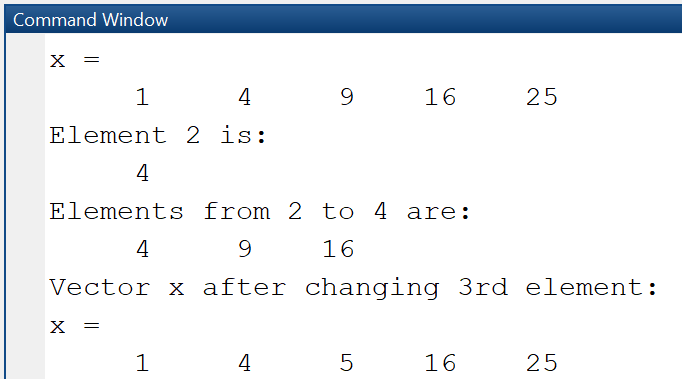Example: Vectors
Find out size and length of vector

Using the whos command, we can see that the vector x is actually a matrix with 1 row and 5 columns, is of class double and uses 40 bytes of storage.

x = [1 4 9 16 25]
disp('Whos x:')
whos x
disp('Size of x:')
sz = size(x)
disp('Length of x:')
n = length(x)
Output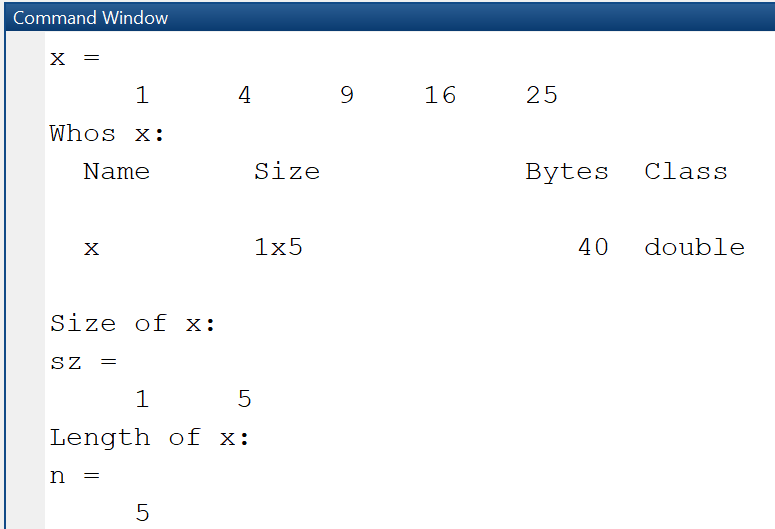Random numbers

An important usecase for creating vectors is that of generating them from random numbers. This can be done using the functions rand, randi, randn. rand creates uniformly distributed random numbers of data type double, by default in the interval (0,1), and depending on the provided arguments, you can generate a matrix, a column or row vector, or a single number. randi creates uniformly distributed random integers, as matrix, column or row vector.

Example: Random numbers
Using rand and randi

% Create 3x5 matrix; random numbers in (0, 1)
r_mat = rand(3, 5)
% Col vector with 5 random elements in (0, 1)
r_col = rand(5, 1)
% Row vector with 5 random elements in (0, 1)
r_row = rand(1, 5)
% Row vector with 5 elements in range (a, b)
a = 10;b = 20;
r_row_10_20 = rand(1, 5)*(b-a) + a
% Create n integer random numbers in [a, b]
a = 10;b = 20; n = 5;
r_int_10_20 = randi([a, b],1, n)
Output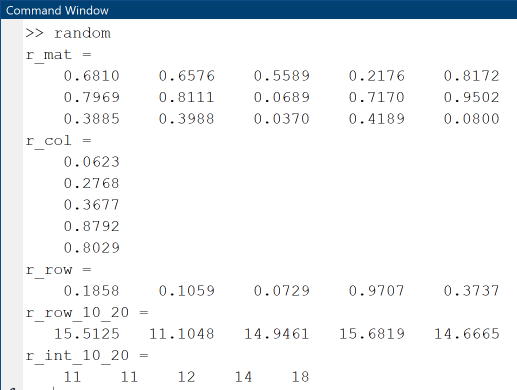Vector operations

MATLAB supports vector operations of two kinds: mathematical operations according to the rules of linear algebra (transposition, addition, multiplication) and array-type elementwise operations. Elementwise operations are distinguished from vector operations by usage of the dot operator (.).
x * y means matrix multiplication, and works only if x is a 1xn row vector and y is a nx1 column vector.
x .* y means elementwise multiplication and works only if x and y are both row (or column) vectors of same size.

Vector and elementwise operations are the same for addition and subtraction, so the operators .+ and .- are not used. Since vectors actually are a special type of matrix, these operations are the same as the described below for matrices.

Example: Vector operations
Create vector with 5 elements

We first create a row vector x with 5 elements, then a row vector y from x by adding 1 to each element, and a third vector z by elementwise multiplication of x and y.

x = 0:2:8 % x = 0,2,4,6,8
% Add 1 to each element
y = x + 1 % y = 1,3,5,7,9
% Multiply x and y elementwise
z = x .* y % z = 0*1+2*3+...+8*9
% Slicing
% Extract a slice from 2 to 5
xslice = x(2:4) % x1 = 2,4,6
Output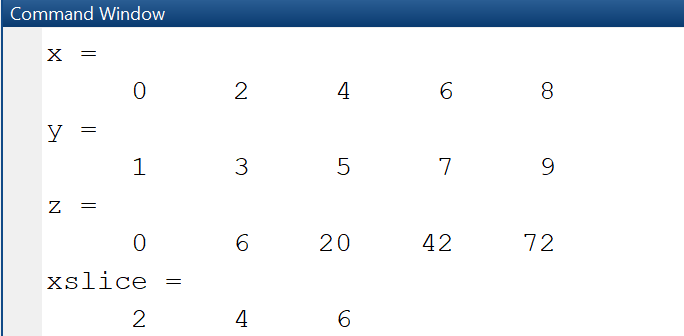Matrices

Data of MATLAB programs are stored in one- and multi-dimensional arrays (e.g. vectors, matrices) if the elements are of same data type, in cell arrays that can contain data of varying types and sizes, or in tables, if they are spreadsheet-like data with columns of same data type. A good understanding of matrices is important, since variables and vectors are actually a special case of matrices. Matrices are created in different ways: by enumerating their elements explicitly in square brackets, using functions, or using for-loops.

Create matrix using enumeration
When declaring a matrix by explicit enumeration, row elements are separated by a space and columns are separated by semicolon.

Example: Create matrix
Create matrix by enumeration

In this example we declare a matrix with two rows and three columns, display it using disp, and find out type and size using whos. The whos-command shows that it is indeed a 2x3 matrix and uses up 48 bytes of memory.

A = [1 2 3;4 5 6]; % 2 rows, 3 cols
disp('Matrix A:')
disp(A)
whos A
OutputCreate matrix using functions
Many mathematical problems require to use matrices initialized with specific values, such as the identity matrix. In MATLAB, the declaration of these matrices is done using MATLAB functions such as zeros - initializes a matrix with all zeros, ones - initializes a matrix with all ones, rand - creates a matrix with random values, or eye - creates the identity matrix.

Example: Create matrix
Create matrices using functions zeros, ones, eye

In this example we create three matrices: A is a matrix with m rows and n columns whose elements are initialized with zeros. B is a mxn matrix whose elements are all initialized with 1. I_n is the identity matrix with n rows and n columns, with ones on the diagonal and 0 on all other elements.

m = 2; n = 3;
% mxn matrix with elements initialized to 0
A = zeros(m, n)
% mxn matrix with elements initialized to 1
B = ones(m, n)
% nxn identity matrix with 1 on diagonal
I_n = eye(n, n)
Output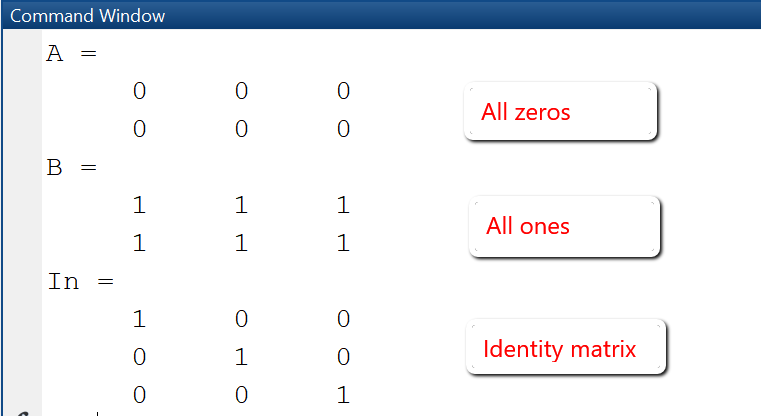Create matrix using for loops
The creation of a matrix using for loops is required when the size and even the element values change dynamically depending on some parameters. In this case, it is recommended to preallocate the maximal required memory using the zeros()-function.

m = 3; n = 4; % m rows and n columns
A = zeros(m, n); % create a mxn matrix initialized with zeros
for i=1:m % loop over the rows
for j=1:n % loop over the columns
A(i,j) = i*j; %
end
end
disp(A)

Elements of a matrix are accessed using a row index and a column index that both start at 1. For example, to access element in row i and column j in a matrix A with m rows and n columns, we write A(i, j). Element with row-index 1 and column-index 1 is accessed with A(1, 1), etc.

Example: Slicing matrices
Extract elements from a matrix

Accessing elements from a matrix is done by using indexing and slicing. In this example we first extract element with row-index 2 and column-index 3 and store in in a variable el23, then we extract the rows from 1 and 3 and all columns and store the result in a new matrix A1, finally we extract the rows from 2 to 3 and columns from 1 to 2 and store the result in a new matrix A2.

A = [1 2 3; 4 5 6; 7 8 9]
el23 = A(2,3) % el23 = 6:
% Extract rows 1 and 3
A1 = A([1,3],:) % A1 = [1 2 3; 7 8 9]
% Extract rows 2 and 3 and and columns 1 and 2
A2 = A(2:3,1:2) % A2 = [4 5; 7 8]
OutputMatrix operations

MATLAB supports matrix operations of two kinds: mathematical operations according to the rules of linear algebra (transposition, addition, multiplication) and array-type elementwise operations. Elementwise operations are distinguished from matrix operations by usage of the dot operator (.): A * B means matrix multiplication, A .* B means elementwise multiplication, A.^2 means that each element of the matrix is taken at power 2, etc. Since matrix and elementwise operations are the same for addition and subtraction, the operators .+ and .- are not used.

Example: Matrix operations

This example shows frequently used matrix operations: transposition, addition, multiplication and elementwise multiplication. The transposed of a matrix A is obtained by writing A', i.e. the matrix name followed by a quotation mark.

% Transpose a matrix
A = [1 2; 3 4]
B = A' % B = [1 3; 2 4]
A = [1 2; 3 4];B = [1 3; 2 4];
C = A + B % C = [2 5; 5 8]
% Matrix multiplication
A = [1 2; 3 4];B = [1 3; 2 4];
D = A * B % D = [5 11; 11 25]
% Elementwise multiplication
A = [1 2; 3 4];B = [1 3; 2 4];
E = A .* B % E = [1 6; 6 16]
OutputFunctions

A function is a reusable piece of code that is executed only when called. It is defined once and can be called multiple times. Functions may have parameters and also may return parameters. The most frequent application of functions is to use predefined MATLAB-functions such as plot (for plotting) or linspace for creating equidistant vectors, or solvers such as ode45. However, when writing larger MATLAB programs, you should structure them using self-defined functions, or, in a more advanced setting, object-oriented programming and classes.
User-defined functions can be defined in multiple ways:

• Global function: Function is stored in a separate m-file.
• Local function: Function is stored inline in the script where it is called.
• Anonymous function: Function is defined as an anonymous function in the script where it is called.

Important: While the variables of a MATLAB script are displayed in the workspace, the variables used in a function are local variables and not visible in the workspace. Global functions must have an unique name, must be on the MATLAB path and can be used in any other script. Local and anonymous functions are visible only in the script in which they have been defined, their name must be unique within the file in which they have been defined.

(1) Function stored in a separate m-file

In most cases, it is recommended to put the code of a function in its own function script. A function script is a MATLAB m-file that contains only the definition of a single function and cannot be executed as a "normal" script. Function and function script must have the same name, for example a function with name myfun1 must be stored in a function script with name myfun1.m. By storing a function in its own script, the function can be used by any other script in the MATLAB path by calling it with specific arguments.

Defining a function
Functions begin with the keyword "function", followed by the statement
outputargs = function_name(inputargs)
and end with the keyword "end". The actual function statements that define the relationship between the input arguments and the output arguments are written in the function body between the first line and the last line. In order to display the general syntax of a function definition and generate a template for a new function, we use the New > Function-Button in the EDITOR-Tab. This creates a new function script "untitled.m" for the function "untitled" with two generic input arguments and two generic output arguments. Starting with this template, we create our own functions by changing name, arguments and code as needed.

function [outputArg1,outputArg2] = untitled(inputArg1,inputArg2)
%UNTITLED Summary of this function goes here
%   Detailed explanation goes here
outputArg1 = inputArg1;
outputArg2 = inputArg2;
end

Example: Functions
Define and call a function myfun1.

In this example, we create a function myfun1 that takes as input an array-parameter x and calculates the function values y = x*exp(-x2). The code to the left shows the function definition in the function script. In the test script myscript1.m we first create an equidistant vector x, then calculate the y-values by calling the function myfun1 and finally create a plot of the so created x,y-values.

Function myfun1.m

function y = myfun1(x)
% Define the function y = x exp(-x^2)
%   Input: x -- a vector
%   Output: y -- a vector (same size as x)
y = x .* exp(-x.^2);
end

Script myscript1.m

x = -5:0.1:5; % Create vector x
y = myfun1(x); % Calculate y
plot (x, y, '+-'); % Plot y vs. x
title('y(x) = x * exp(-x^2)');
xlabel('x'); ylabel('y');
Output

MATLAB's plot-function is used to create 2D-line plots of data given as (x,y)-values. The plot-function takes as input two vectors that must be of same size, plus a number of formatting options for specifying line type, color, width, title, legend, axis labes etc.(2) Local functions

Functions that will be used only in one script can be stored as local functions inline in that script, and should be placed at the end of the script. Local functions are visible only in the script in which they have been defined and cannot be used in other functions. Why use local functions at all, since the primary goal of a function is to make it reusable? There are situations when you develop new solvers for numerical methods and program variants of the same function, so you need to use it only locally in a test setting and also want to reuse the function name.

Example: Local functions
Define and plot two functions

In this example, we create two functions myfun1 and myfun2 inline in the test script "test_localfunction.m". Both functions myfun1 and myfun2 have as input parameter a vector x, and calculate the y-values for given mathematical functions.

% test_localfunction.m
x = -1:0.1:1;
plot(x, myfun1(x), '+-');
hold on
plot(x, myfun2(x), '+-');
legend('myfun1','myfun2');
title('Line-plot of two functions');
xlabel('x'); ylabel('y');
function y = myfun1(x)
y = sin(x) .* exp(-x.^2);
end
function y = myfun2(x)
y = cos(x) .* exp(-x.^2);
end
Output

In line 4 we used the command hold on so that MATLAB displays both plots on the same figure.(3) Anonymous functions

An anonymous function is a function that is not stored in a script file, instead it is associated with a variable of type function_handle. Informally, it is an inline function that is written in just one line with the help of the @-symbol. Anonymous functions are used for functions whose defining statement can be written in just one line.
General syntax is <function_name> = @ (<param_list>) ( <computation>).

Example: Use anonymous functions
In this example three mathematical functions are defined as anonymous functions.

• Line 1-3: Define function f(x) = sin(2πx) and then calculate the function values at points x = 1 and x = 2.
• Line 4-8: Define polynomial p(x) = 1 + 2x + x2 and then calculate the function values at the equidistant points x = [0, 0.5, 1.0, 1.5, 2.0]. Since in the definition of the polynomial the dot-operator is used, it is allowed to pass a vector as argument to the function.
• Line 8-11: Define a function of two variables u(x,y) = 25xy, where x and y are allowed to be vectors of same size. Then, create two vectors x, y using linspace and calculate the function values z for these vector arguments.
% Example 1: Sin-Function
f = @(x) sin(2*pi*x);
y1 = f(1); y2 = f(2);
% Example 2: Polynomial
p = @(x) (1 + 2.*x + x.^2);
x = 0:0.5:2; % x = [0, 0.5, 1.0, 1.5, 2.0]
y = p(x);
% Example 3: Function with two variables
u = @(x,y)(25.*x.*y);
x = linspace(0,1,10); y = linspace(0,2,10);
z = u(x, y);

Plotting in MATLAB

When solving a mathematical problem such as an ordinary or partial differential equation numerically, it is important to be able to visualize the solution and the intermediate steps quickly and effortlessly, as this helps the intuitive understanding of the physical problem. MATLAB has a number of functions for creating different types of 2D and 3D plots: plot, histogram, surf, mesh, contour, quiver etc., that make this an easy task.

Here we explore the plot-functionality more in detail. Firstly, all plots are organized in figure windows, that are created with the figure command. If the command figure is not specified, a figure is created by default. The figure-command allows to specify options for the creation of the figure window, such as position and title.

2D-Plots with plot

The simplest plot is a 2D-line-plot of a function y = f(x), using the function plot(). The plot-function has the general syntax plot(X,Y), that creates a 2D line plot of the data in Y versus the corresponding coordinate values in X. X and Y must be vectors or matrices with matching size. For example, in order to create a plot of y = f(x) = x^2 for the discrete values X = [1,2,3,4,5] and Y = [1,4,9,16,25], we write

plot([1,2,3,4,5], [1,4,9,16,25])

or simply

plot([1,4,9,16,25])

Advanced usage of plot() enables to use other input parameters and specify options specified as name-value-pairs.

Example 1: 2D-Line-Plot

In the next example we explore some of the plot options and display the sin and cos-function on the same axis.

• Line 2: Create a discretization of the interval [-2*pi, 2*pi] using an equidistant vector with 51 data points.
• Line 3 and 4: Define the y-values for the functions to be plotted.
• Line 5: Create new figure with width 700, heigth 500 at position (50, 50).
• Line 6: Plot the sin-function given by the coordinate vectors x and y1.
• Line 7: hold on retains plots in the current axes so that new plots can be added
• Line 8: Plot the cos-function given by the coordinate vectors x and y2.
• Line 9-11: Specify a title, legend and labels for the axes.
Example: 2D-Line-Plot
Plot functions on same axis using hold on.
clear all;clc;format compact;
x = linspace(-2*pi, 2*pi, 51);
y1 = sin(x);
y2 = cos(x);
figure('Position', [50,50,700,500]);
plot (x, y1, '-s', 'LineWidth',1);
hold on
plot (x, y2, '-o','LineWidth',1.5);
title('Plot of the sin- and cos-function');
legend('y1 = sin(x)', 'y2 = cos(x)');
xlabel('x'); ylabel('y');grid on;
FigureIn MATLAB, it is possible to display plots on the same figure but on different axes using the subplot-function. The command subplot(m, n, p) divides the current figure in a grid of plots as specified by its parameters: m rows, n columns and the next plot at position p.

Example 2: 2D-Plot using figure and subplots

Assume you need to plot the functions y1(x) = sin(x)*exp(x) and y2(x) = x*cos(x) on the same figure, so that the first plot is above the second plot. Then with the command subplot(2,1,p) you create a grid with 2 rows and 1 column, plus new axes at position p = 1,2, as in this example.

• Line 5: Create subplot for upper plot at position p = 1
• Line 6-9: Create plot that will be placed at subplot p = 1. We specifiy linewidth, color and marker edge color of the plot using name-value pairs. Code line 6 is split using ellipses (...).
• Line 10: Create subplot for lower plot at position p = 2
• Line 11-14: Create plot that will be placed at subplot p = 2.
Code: Plot with subplots
Plot functions in a 2x1 grid using subplots
x = linspace(-2*pi, 2*pi, 41);
y1 = sin(x).*exp(x);
y2 = cos(x).*x;
figure('Position', [50,50,700,500]);
subplot(2,1,1)
plot (x, y1, '-s', 'LineWidth',1.1, 'Color','red', ...
'MarkerEdgeColor','red');
title('y(x) = sin(x)*exp(-x) ');
xlabel('x'); ylabel('y');grid on;
subplot(2,1,2)
plot (x, y2, '-o','LineWidth',1.2, 'Color','black', ...
'MarkerEdgeColor','black');
title('y(x) = cos(x)*x');
xlabel('x'); ylabel('y');grid on;
Figure: Plot with subplots
Plot functions in a 2x1 grid using subplots3D-Plots with surf

For creating a 3D-surface-plot of a function z = u(x,y), the function surf() is used. The surf-function has the general syntax surf(X, Y, Z), that creates a 3D height plot of the data in Z versus the coordinate values in X and Y. X, Y and Z must be matrices with matching size. The two matrices X and Y for the grid are obtained from the coordinate vectors for the x and y axis using meshgrid. If x is a vector with m elements and n is a vector with n elements, meshgrid(x, y) creates two nxm matrices X and Y: X replicates x as row vector n times, and Y replicates y as column vector m times.

The next example shows the basic usage of meshgrid and surf and the involved matrices X, Y, Z.

Example 1: 3D-Plot with surf

In this example, we create a surface plot of z = u(x, y) = x^2 - y^2 for x- and y-coordinates given as vectors.

• Line 1-2: Create coordinate vectors x and y.
• Line 1-2: Coordinate vectors x and y are used as input to create the matrices X and Y with meshgrid().
• Line 4: Calculate 3x4 matrix Z containing the function values.
• Line 5: Plot surface using surf.
Code: 3D-Plot with surf
Plot function u(x, y) = x^2 - y^2
x = [1, 2, 3, 4];
y = [0, 0.5, 1];
[X,Y] = meshgrid(x,y)
Z = X.^2 - Y.^2
surf(X, Y, Z)

The output in the command window is as shown:

Output X, Y from meshgrid:
X =
1     2     3     4
1     2     3     4
1     2     3     4
Y =
0         0         0         0
0.5000    0.5000    0.5000    0.5000
1.0000    1.0000    1.0000    1.0000
Function values Z:
Z =
1.0000    4.0000    9.0000   16.0000
0.7500    3.7500    8.7500   15.7500
0    3.0000    8.0000   15.0000
Figure: 3D-Plot with surf
Plot function u(x, y) = x^2 - y^2Example 2: 3D-Plot with surf

We plot the same function u(x,y) = x^2-y^2 over a rectangular area [a, b] x [c, d] and enhance the plot with details: title, axis labels, colorbar. We also show the contours and gradient vector field using the functions contour and quiver. The gradient of a function u(x,y) at a point is the directional derivative of the function; the gradient at all points of the domain defines a vector field that can be plotted using the quiver-function.

• Line 1: Define u as anonymous function.
• Line 2-3: Define rectangle [a, b] x [c, d] and number of data points nx= 10 and ny = 20 for the discretization.
• Line 4: Create evenly spaced x and y-coordinate vectors.
• Line 5: Generate the grid matrices X, Y from the evenly space x- and y-coordinates.
• Line 6: Calculate matrix Z containing the function values.
• Line 7: Calculate the gradient [px, py] of the function
• Line 9-13: Create surface plot of the function u.
• Line 14-20:Create 2D-plot of function with contour lines and gradient vector field.
Code: 3D-Plot with surf, contour, quiver
Plot function u(x, y) = x^2 - y^2, its contour and gradient
u = @(x,y) x.^2 - y.^2;
a = -5; b = 5; nx = 10;
c = -10; d = 10; ny = 20;
x = linspace(a,b,nx+1);y = linspace(c,d,ny+1);
[X,Y] = meshgrid(x,y);
Z = u(X,Y);
figure('Position', [50,50,700,500]);
subplot(2,1,1)
surf(X, Y, Z);
xlim([a,b]);ylim([c,d]);view(3);
title('Function z = u(x,y) = x^2-y^2')
xlabel('x');ylabel('y');zlabel('z = u(x,y)');
subplot(2,1,2)
contour(X,Y,Z);
hold on
quiver(X, Y, px, py);
xlabel('x');ylabel('y');xlim([a,b]);ylim([c,d]);
colorbar;colormap('jet')
Figure: 3D-Plot with surf, contour, quiver
Plot function u(x, y) = x^2 - y^2, its contour and gradientSymbolic computations

The Symbolic Math Toolbox is used to analyze and solve mathematical problems analytically, for example to find derivatives, partial derivatives and integrals of function expressions, to solve equations, or to plot functions. The symbolic mode is started by typing syms and the name of the symbolic variables, for example:

syms x
syms x y
syms x y z
You can do this interactively in the command window or in a script. Derivative, integral and gradient of functions are calculated symbolically using the functions diff, int and gradient. For symbolic plots we can use a number of functions such as fplot, fsurf and fcontour, their name starts with "f" and they all work on symbolic function expressions.

Code: Symbolic computation
Calculate derivative and integral of functions

The following example shows how to declare functions of one variable and calculate the derivative using diff and integral using int. Note that the symbolic mode is started in line 1 with the syms keyword and the name of the variable x, and then in line 7 with the syms keyword an the name of the function g(x).

syms x
% Declare symbolic function f depending on x
f = sin(x^2)*sqrt(x)
% Determine derivative of f using diff
df = diff(f)
% Declare symbolic function g depending on x
syms g(x)
g(x) = log(x)
% Determine integral of the function g
intg = int(g)
Output
Result of symbolic computation

The usage of symbolic computations is helpful when you develop a new numerical method and need to cross-check the numerical solutions with the exact analytical solutions.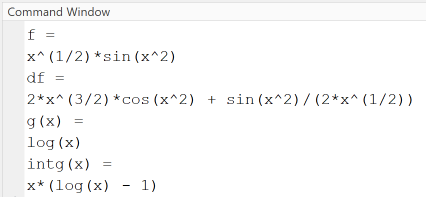MATLAB Quizzes

Test your basic understanding of MATLAB Development Environment and language syntax by taking the MATLAB Quizzes . Each quiz consists of ten questions, each with three to four possible answers, and there may be multiple correct or incorrect answers. Check those answers that you think are correct. If you have answered at least 50% of the answers correctly, you are prepared to take MATLAB a step further, for example by taking the course "Numerical Methods". Have fun!

Tools & References

This tutorial gives an introduction to MATLAB basic syntax. Starting here, there is much more to be learned and done. Further interesting topics are: write MATLAB LiveScripts, use the GUI Editor to create graphical user interfaces, write larger programs using classes and inheritance, develop your own toolbox.

1. MATLAB Help: https://de.mathworks.com/help/matlab/
2. MATLAB Language Fundamentals: https://de.mathworks.com/help/matlab/language-fundamentals.html
3. Octave Software: https://www.gnu.org/software/octave/
4. Octave Manual: https://octave.org/doc/v6.1.0/
5. This Tutorial as PDF-File: MATLAB Tutorial - First steps in MATLAB _ Prof. E. Kiss, HS KL.pdf